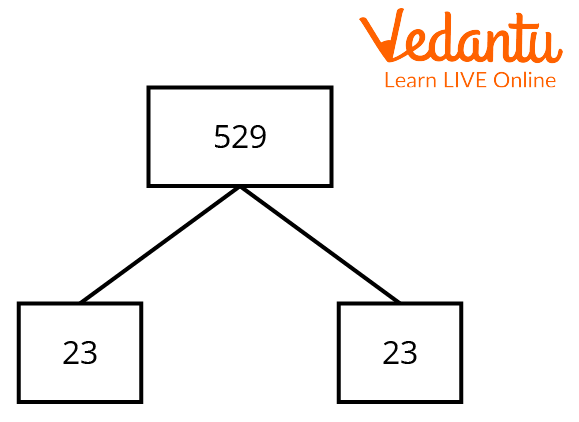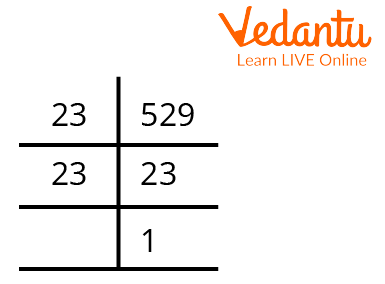Courses
Courses for Kids
Free study material
Free LIVE classes
More

# Finding the Factors of 529 with Solved ExamplesLIVE
Join Vedantu’s FREE Mastercalss

## Factors of a Number: A Brief Introduction

Factors of a number are an important concept in Mathematics. A factor of a number is an integer that divides the given number exactly. The factor when divided by the given number leaves no remainder. There are various methods of finding the factors of a number, namely the factor tree method, prime factorisation method, or simple division method.

Every number has some factors. A number with factors only 1 and itself is called a prime number. On the other hand, a number with more than two factors is called a composite number.

## Factors of 529

The factors of 529 are those numbers that when divided by 529 leave no remainder. This implies that the factors are those numbers that divide 529 exactly.

## Division Method

This is the simplest method to evaluate the factors of 529. In this method, we need to simply think of all the numbers less than 529 that divide 529 and leave the remainder 0.

1 is a factor 529 as

$529 \div 1 = 529\;$, Remainder = 0

23 is a factor of 529 as

$529 \div 23 = 23\;$, Remainder = 0

529 is a factor of 529 as

$529 \div 529 = 1\;$, Remainder = 0

These are the only numbers between 1 and 529 that divide 529 exactly.

Therefore, the factors of 529 are 1, 23, and 529.

## Factor Tree Method

The factor tree method is like creating branches of factors of a given number. Each branch reduces to form a factor of the number. The numbers obtained at the end of the factor tree together form the prime factorisation of the given number as they are all prime numbers.Factor Tree of 529

## Prime Factorisation of 529

Prime factorisation involves factorising or dividing the given number into prime numbers. Prime numbers are numbers that have only two factors, namely 1 and itself. Therefore, while finding the prime factorisation of 529, we think of prime numbers that divide 529.Prime Factorisation of 529

The prime factorisation of 529 is $529 = 23 \times 23 = {23^2}$

## Pair Factors of 529

Pair factors are a pair of integers that when multiplied provide us the product as the given number. We must keep in mind that pair factors can also include negative integers. There are both positive and negative pair factors of 529. This is because the product of two negative integers is always positive.

### Positive Pair Factors

• (1,529) is a pair factor of 529 as $1 \times 529 = 529$

• (23,23) is a pair factor of 529 as $23 \times 23 = 529$

### Negative Pair Factors

• (-1,-529) is a pair factor of 529 as $-1 \times -529 = 529$

• (-23,-23) is a pair factor of 529 as $-23 \times -23 = 529$

Therefore, the pair factors of 529 are listed in the following table:

 Positive Pair Factors Negative Pair Factors (1,529) (-1,-529) (23,23) (-23,-23)

## Square Root of 529

The prime factorisation of 529 also helps us to calculate its square root along with its factors.

• Step 1: To evaluate the prime factorisation of 529

$529 = 23 \times 23 = {23^2}$

• Step 2: Form pairs of numbers occurring twice. In the prime factoridsation of 529, 23 occurs twice.

• Step 3: The square root will be obtained by writing all the numbers in pairs only once.

$\sqrt {529} = \sqrt {23 \times 23} = 23$

The square root of 529 is 23.

## Interesting Facts

• Every number has at least 2 factors which are 1 and the number itself.

• 529 is a composite number as it has more than 2 factors.

• The largest prime factor of 529 is 23, which is also its square root.

• 529 has only one prime factor namely 23.

## Solved Examples

1. Find the product of all the factors of 529.

Solution: The factors of 529 are 1, 23, and 529.

The product of the factors is $1 \times 23 \times 529 = 12167$

2. Is 24 a factor of 529?

Solution: We can check whether 24 is a factor of 529 by dividing 529 by 24. If the remainder after division is 0, then it will imply that 24 is a factor of 529.

$529 \div 24 = 22\dfrac{1}{{24}} \\ 529\; = \;22 \times 24 + 1$

This implies 24 leaves a remainder of 1 when divided by 529.

Therefore, 24 is not a factor of 529.

3. Find the common factors of 529 and 23.

Solution: The factors of 529 are 1, 23, and 529.

Since 23 is a prime number, it has only two factors, which are 1 and the number itself. The factors of 23 are 1 and 23. Hence, the common factors of 23 and 529 are 1 and 23.

## Practice Questions

1. How many factors does 529 have?

1. 5

2. 4

3. 2

4. 3

Ans: Option D

2. What is the sum of the products of 529?

1. 453

2. 553

3. 653

4. 753

Ans: Option D

## Conclusion

The article highlights the process of obtaining the factors of 529. These factors can be calculated using two straightforward but critical methods which are the division method and the factor tree method. The factors of 529 are 1, 23, and 529. The prime factorisation of 529 is $23 \times 23 = {23^2}$. It not only helps us calculate the prime factors of 529 but also enables us to establish the square root of 529 which is 23.

Last updated date: 20th Sep 2023
Total views: 110.1k
Views today: 3.10k

## FAQs on Finding the Factors of 529 with Solved Examples

1. By which numbers is 529 divisible?

The numbers which can be divided by 529 are those numbers that do not leave any remainder. We can also say that 529 is exactly divisible by these numbers. 529 will be a multiple of these numbers which means 529 comes in the multiplicative table of these numbers. These numbers are called the factors of 529. Since the prime factorisation of 529 is 529 = 23 23 = 232, the factors of 529 are 1, 23, and 529. Therefore 529 is exactly divisible by 1, 23, and 529.

2. Is 529 a perfect square?

A perfect square is a number that is obtained by multiplying a number with itself. When 2 is multiplied by 2, we get 4. Therefore, 4 is a perfect square. Some other examples of a perfect square are 9, 36, 81, etc. To find whether 529 is a perfect square, we first need to check its prime factorisation. The prime factorisation of 529 is 529 = 23 23 = 232. This implies that when 23 is multiplied by 23, we get 529. Therefore, 529 is a perfect square number and it is the square of 23.

3. What are the factors of 529 in pairs?

When we write the factors in pairs such that the two members when multiplied result in the given number, such pairs are called pair factors. We can list down the pair factors of 529 as well. This involves pairs of either both positive or negative integers. The pair factors of 529 are (1,529), (23,23), (-1,-529) and (-23,-23). All these pairs when multiplied give us 529, hence they are called pair factors.